# Mathematics

Origin’s powerful mathematics tools can be categorized in the following groups:

## Data Transformation

### Column and Cell Calculations

Simpler Column/Cell Notation for Column/Cell Formulas

• Auto- column short naming as columns are added, deleted or moved.
• Column formula notation is similar to Excel or Google Sheets, as for instance ...
• B, C - column B and C
• B1 - 1st cell in column B
• 2!B - column B of sheet 2

Cell Formula

• Stretch and extend formula vertically/horizontally
• Fixed cell referencing is supported
• Use script-accessible functions and/or custom expressions
• Results update when source cells change, or with other changes such as data filter

F(x) Column Formula row

The F(x) Column Formula row in Origin worksheet lets you directly type expressions to calculate column values based on data in other columns and metadata elements

Set Column Values Dialog

Besides F(x)= column label row in worksheet to directly enter formula, Set Column Values dialog offers more useful features:

• Calculations can involve columns from other worksheets
• Over 500 functions in categories such as math, engineering, statistics, date-and-time, and string processing
• Before Formula Script to pre-process data prior to calculating results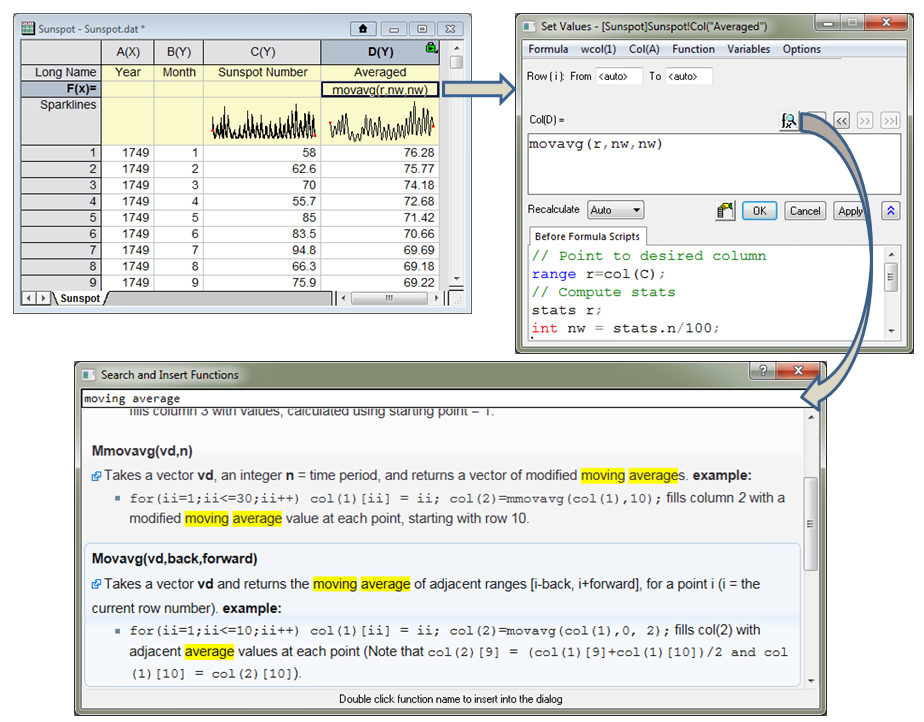The F(x) Column Formula row in Origin worksheet lets you directly type expressions to calculate column values based on data in other columns and metadata elements. The expression can be further edited in the Set Values dialog which provides a lower panel to execute Before Formula scripts for pre-processing data. The Set Values dialog also provides a search button to quickly find and insert functions from over 500 built-in functions. User-defined functions can also be added for custom transforms.Assign formula to User-defined Parameter Rows to easily set up calculations such as calculating mean and std. deviation as in this example. Enter formula in one cell and drag-and-extend to the right to other cells in adjacent columns.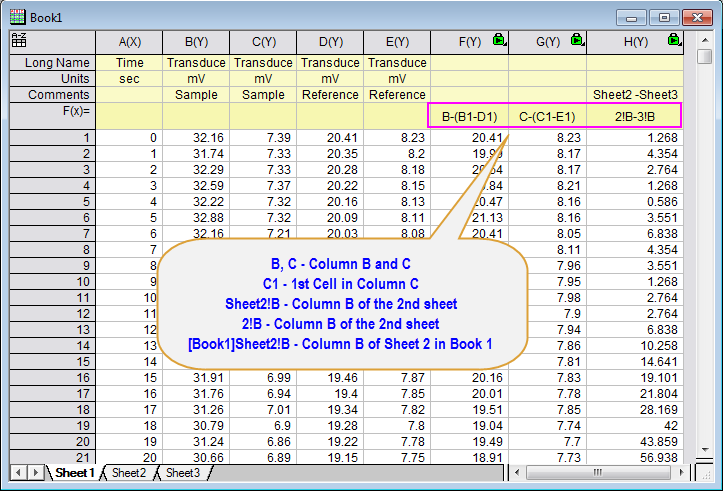Excel-like spreadsheet cell notation to be used in defining column formulas. Whenever this notation system is turned on for a workbook, a small A to Z icon will appear in the upper left hand corner of the workbook. With this notation, the column short names follow alphabetic orders and are not renamable. Column Formulas have now been significantly shortened and can be written in a similar fashion to Excel and Google sheets

### Normalization

Origin provides a tool for performing normalization of data either from a worksheet or a graph. The tool provides various methods for normalizing data.

• Divided by a Specified Value
• Normalize to [0, 1]
• Normalize to [0, 100]
• Z Scores (standardize to N(0, 1))
• Divided by Max
• Divided by Min
• Divided by Mean
• Divided by Median
• Divided by SD
• Divided by Norm
• Divided by Mode
• Divided by Sum
• Use Reference Plot\Use Reference Column
• Divided by Reference Cell
• Normalize to Point Picked from Graph

### Average Multiple Curves

The Average Curves tool enables you to average multiple curves, even when the curves have different X values. The standard deviation or standard error can also be output to be set as error bars for plotting.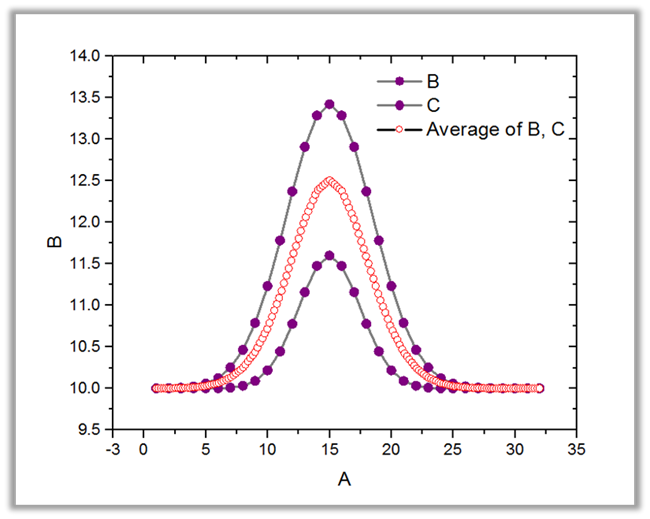The Average Curves tool lets you average multiple curves. Curves with different X values is also supported.

### Simple Math Calculations on Column or Curves

Origin provide tools to perform five simple math operations on columns or on curves. The tool also work for curves with different X values.

• Subtract
• Divide
• Multiply
• PowerThe Simple Math tool enable you to perform simple math between curves. Data with different X ranges or X spacing is also supported.

## Interpolation and Extrapolation

### 1D Interpolation/Extrapolation

Interpolation/extrapolation

Generate data with uniformly-spaced X values

Interpolate/Extrapolate Y From X

Generate data with a set of given X values

Trace Interpolation

Appropriate for cyclic or periodic curveCompared to ordinary interpolation, trace interpolation follows the sequence of the data points, and is therefore more appropriate for cyclic or periodic data. This example shows the difference between ordinary interpolation and trace interpolation on the same periodic data.

### 2D Interpolation/Extrapolation

2D Interpolation/Extrapolation

Generate data with uniformly-spaced XY datasets

Interpolate Z From XY

Generate Z values for a set of given XY values

XYZ Trace Interpolation

Perform interpolation of XYZ data by following the data trace in 3D.

### 3D Interpolation

Perform interpolation on (X, Y, Z, F) data points, and generate data with uniformly-spaced datasets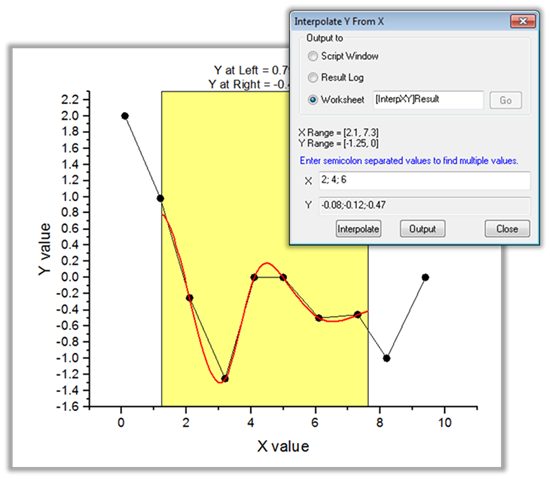The Interpolation Gadget in Origin allows for easy up-or-down sampling of existing data over a selected range of X values.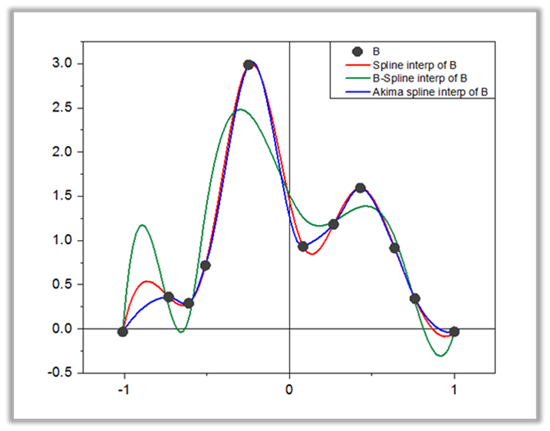The Interpolate tool supports four interpolation methods: Linear, Cubic Spline, Cubic B-Spline and Akima SplineThe Interpolate/Extrapolate Y From X tool lets you start from an XY dataset or curve, and generate Y values for a list of specific X values. The specific X values can be non-uniformly spaced. Four interpolation methods are provided: Linear, Cubic Spline, Cubic B-Spline and Akima SplineThe 2D Interpolation/Extrapolation tool enables interpolation or extrapolation on uniformly spaced XY dataset with five methods: Nearest, Bilinear, Bicubic, Spline and Biquadratic. Origin also support Interpolate Z from XY tool to find Z values at a given set of XY values.

## Differentiation and Integration

### Differentiation

The differentiation tool in Origin can calculate derivative up to 9th order. Option for smoothing is also available for handling noisy data.The Differentiate Gadget also enables you to view the results interactively in a separate graph.

### Integration

• Numerical Integration on Columns
• 2D Integration Gadget and Volume Integration PRO

### Area Calculation

• Polygon Area
• Surface Area Calculation PRO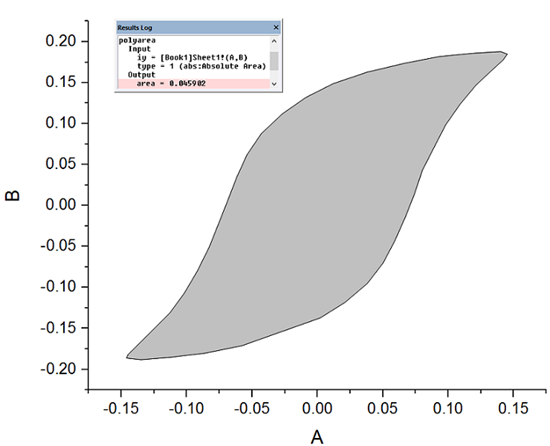The polyarea tools can be used to compute the (signed) area of a non-self-intersecting polygon in XY plane.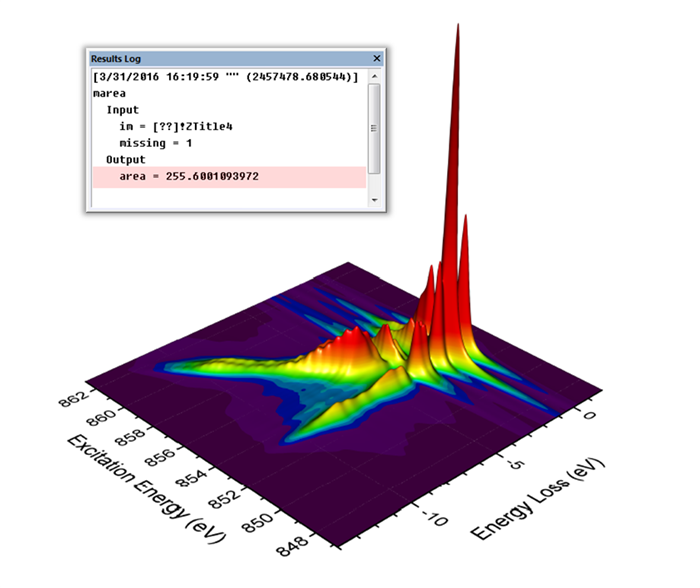The marea and xyzarea tools enable you to calculate the area of surface plotted from either matrix or XYZ data.The Differentiate Gadget lets you specify a desired derivative order and view the results interactively in a separate graph.The Integrate Gadget provides an easy way to perform area calculations over a desired X range.The tool provides various baseline options including the option to use another curve as baseline, thus allowing for calculating area between two curves.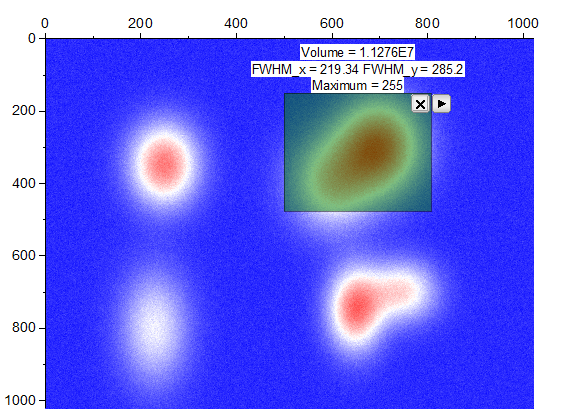The 2D Integration Gadget can perform volume integration over a specified XY region. The tool also provides options for baseline correction.

## Matrix-Based Operations

For mathematical operation on matrix data, the Set Matrix Values dialog also allows Before Formula Script to pre-process data prior to calculating the formula. Origin also provides tools for calculating the inverse of a matrix, shrink or expand a matrix, or filter a matrix using built-in or custom filters.

 Set Matrix Values Inverse Matrix Shrink/Expand Custom Filter Median Filter Special FilterRaw signal and noise are imported into 1st two matrix objects in one matrix sheet. A 3rd matrix object is added and calculated by subtracting noise from raw signal. The green lock indicates this 3rd matrix is an output of an operation so if raw signal or noise data changes, it can recalculate. Thumbnail images are turned on to show the image preview of data.